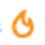# 0 division help

Let x be any real no.

Then, if we do as follows:

$\frac { 0 }{ x }$ , we get 0 as only $x\times 0$ is 0

If we do $\frac { x }{ 0 }$ it is indeterminate or not defined.(I know the reason)

But if we do $\frac { 0 }{ 0 }$ we say the answer is 0. Is it really 0 or indeterminate because 0* any no. is 0 so should'nt the answer be not defined.Note by Satyajit Ghosh
6 years, 11 months ago

This discussion board is a place to discuss our Daily Challenges and the math and science related to those challenges. Explanations are more than just a solution — they should explain the steps and thinking strategies that you used to obtain the solution. Comments should further the discussion of math and science.

When posting on Brilliant:

• Use the emojis to react to an explanation, whether you're congratulating a job well done , or just really confused .
• Ask specific questions about the challenge or the steps in somebody's explanation. Well-posed questions can add a lot to the discussion, but posting "I don't understand!" doesn't help anyone.
• Try to contribute something new to the discussion, whether it is an extension, generalization or other idea related to the challenge.

MarkdownAppears as
*italics* or _italics_ italics
**bold** or __bold__ bold
- bulleted- list
• bulleted
• list
1. numbered2. list
1. numbered
2. list
Note: you must add a full line of space before and after lists for them to show up correctly
paragraph 1paragraph 2

paragraph 1

paragraph 2

[example link](https://brilliant.org)example link
> This is a quote
This is a quote
    # I indented these lines
# 4 spaces, and now they show
# up as a code block.

print "hello world"
# I indented these lines
# 4 spaces, and now they show
# up as a code block.

print "hello world"
MathAppears as
Remember to wrap math in $$ ... $$ or $ ... $ to ensure proper formatting.
2 \times 3 $2 \times 3$
2^{34} $2^{34}$
a_{i-1} $a_{i-1}$
\frac{2}{3} $\frac{2}{3}$
\sqrt{2} $\sqrt{2}$
\sum_{i=1}^3 $\sum_{i=1}^3$
\sin \theta $\sin \theta$
\boxed{123} $\boxed{123}$

Sort by:

Any number divided by $0$ is undefined.

Dividing by a certain number is the same as multiplying by its multiplicative inverse.

The multiplicative inverse of $0$ is not defined. You can try to define it, but you're going to run into inconsistencies. You can try to patch those inconsistencies by giving $0^{-1}$ more properties. Then you're going to run into other inconsistencies. Finally you may be able to do it, but it won't be of much use.

Some things are best left undefined.

- 6 years, 11 months ago

So you mean 0/0 is undefined. Right?

- 6 years, 11 months ago

Yes, it is.

- 6 years, 11 months ago

Thanks

- 6 years, 11 months ago

What a lame doubt

- 6 years, 11 months ago

- 6 years, 10 months ago

Who says 0/0 is 0. That person is mad. Of course it is undefined

- 6 years, 11 months ago

what is the value if x/0. find

- 6 years, 10 months ago

Its undefined.

- 6 years, 10 months ago

means of undefined.

- 6 years, 10 months ago

It means that you cannot determine the answer.

As x * any no. cannot give x

- 6 years, 10 months ago

Hmm the answer should be not defined because 0/0 can also be equal to 1 like 2/2,3/3 are equal to 1 and it can also be 0 because 0 has no value ,so the answer can't be defined ^_^

- 5 years, 8 months ago